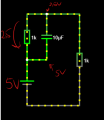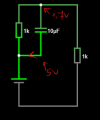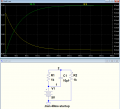# The voltage on capacitor.

#### Xenon02

Joined Feb 24, 2021
355
Hello !

I've got more simpler question.

I've got something like this :Do you maybe know why the capacitor stopped discharging ? I mean there is still voltage difference on one side there is 5V and on the other side there is 2,5V.

Here :He was still discharging because there is 5V and 1,7V on two sides.

#### WBahn

Joined Mar 31, 2012
26,896
For a moment, consider the circuit with the capacitor removed. There will be 2.5 V across each resistor, right?

Now imagine charging the capacitor to 2.5 V somewhere else and disconnecting it. It will still have 2.5 V across it, right?

Now imagine connecting this charged capacitor across the resistor in the circuit. There's no reason for the charge on the capacitor to change, so no current flows into or out of it. There is still current flowing in the resistors.

Current flow in a capacitor is not a function of the voltage across it, but rather the rate of change in that voltage. If the voltage across it is unchanging, then the capacitor's charge won't change.

#### Xenon02

Joined Feb 24, 2021
355
For a moment, consider the circuit with the capacitor removed. There will be 2.5 V across each resistor, right?

Now imagine charging the capacitor to 2.5 V somewhere else and disconnecting it. It will still have 2.5 V across it, right?

Now imagine connecting this charged capacitor across the resistor in the circuit. There's no reason for the charge on the capacitor to change, so no current flows into or out of it. There is still current flowing in the resistors.

Current flow in a capacitor is not a function of the voltage across it, but rather the rate of change in that voltage. If the voltage across it is unchanging, then the capacitor's charge won't change.

I thought that when there is a difference in both sides of capacitor like 5V and 1,7V. Then the capacitor will be discharging.

Of course I understand what do you mean here. I mean I thought that the capacitor will still be discharging because there is a voltage difference right ? On the one side there is 5V and on the other 2,5V. So theoretically I should be discharging. Like in the one picture where there was 5V on one side and 1,7V on the other. And it was discharging.

#### WBahn

Joined Mar 31, 2012
26,896
It isn't discharging because there if 5 V on one side and 1.7 V on the other. The voltage is changing because there is current flowing through it.

What is the current flowing through the resistor that is across the capacitor? It's (5 V - 1.7 V) = 3.3 V. That voltage appears across a 1 kΩ resistor, so there are 3.3 mA of current flowing in that resistor.

What about the other resistor? It has 1.7 V across it and since it is also 1 kΩ, it only has 1.7 mA of current. That means that the remaining 1.6 mA of current has to be coming out of the capacitor, which is causing it to discharge. As it discharges, the voltage across the resistors change, meaning that the currents in them change. Eventually, the voltage across the capacitor is such that the current flowing in the two resistors is the same and no more current is flowing in the capacitor. With no current flowing through it, it can neither charge nor discharge, and so the voltage across it remains the same.

Change the value of the resistor across the capacitor to 4 kΩ. Now the balanced condition is when the voltage across the capacitor is 4V, resulting in 1 mA flowing in each resistor. Then change that resistor to 0.25 Ω and you will see that the balance point will be 1 V across the capacitor with 4 mA flowing in each resistor.

#### Xenon02

Joined Feb 24, 2021
355
I thought that current flows from high voltage to low voltage and I thought the same work with capacitor and because there was 5V on one side and 2,5V on the other side then the current would flow.

But maybe I was wrong. But I guess I understand it.

#### WBahn

Joined Mar 31, 2012
26,896
I thought that current flows from high voltage to low voltage and I thought the same work with capacitor and because there was 5V on one side and 2,5V on the other side then the current would flow.

But maybe I was wrong. But I guess I understand it.
This is like saying that water flows a higher level to a lower level. It's a nice way to keep a basic concept in mind, but there are limits to all things. Set a bucket of water on top of the counter and the water doesn't flow to the floor just because it's at a higher level. There needs to be an available path for it to flow.

The same with a capacitor. How a capacitor works is that you have two conductors separated by an insulator such that electrons can't flow completely through the device. Instead, when an electron enters one side it gets deposited on the plate on that side and essentially pushes an electron off the plate on the other side so that a different electron leaves. As far as the circuit is concerned, an electron is an electron, so it might as well have been an electron that flowed in one terminal and out the other.

But in actuality, there is now an excess electron on one plate and a deficit of an electron on the other. The result is an electric field between the plates that would like to undo this, if possible, by creating an voltage across the capacitor plates.

So in your circuit when there is 2.5 V across the capacitor but no current is flowing through it, what has happened is that enough electrons have build up on one plate (and taken from the other) such that, within the capacitor, there is 2.5 V across the gap between the plates that results in no current flow through it.

•Harry Trietley

#### Xenon02

Joined Feb 24, 2021
355
This is like saying that water flows a higher level to a lower level. It's a nice way to keep a basic concept in mind, but there are limits to all things. Set a bucket of water on top of the counter and the water doesn't flow to the floor just because it's at a higher level. There needs to be an available path for it to flow.

The same with a capacitor. How a capacitor works is that you have two conductors separated by an insulator such that electrons can't flow completely through the device. Instead, when an electron enters one side it gets deposited on the plate on that side and essentially pushes an electron off the plate on the other side so that a different electron leaves. As far as the circuit is concerned, an electron is an electron, so it might as well have been an electron that flowed in one terminal and out the other.

But in actuality, there is now an excess electron on one plate and a deficit of an electron on the other. The result is an electric field between the plates that would like to undo this, if possible, by creating an voltage across the capacitor plates.

So in your circuit when there is 2.5 V across the capacitor but no current is flowing through it, what has happened is that enough electrons have build up on one plate (and taken from the other) such that, within the capacitor, there is 2.5 V across the gap between the plates that results in no current flow through it.
I understand the concept it's just that I thought that current always goes from high voltage to low.

But maybe this is not true for everything.

#### crutschow

Joined Mar 14, 2008
30,455
I understand the concept it's just that I thought that current always goes from high voltage to low.
For a resistor, yes.
For a capacitor, not after it has charged to the applied voltage.
A capacitor cannot pass a steady DC current once it is charged, somewhat like filling a bucket with water until it is full.

#### Xenon02

Joined Feb 24, 2021
355
So how do I know when is it charging and when not ?

If not the voltage difference which for example was here 5V and 2,5V then what ?

#### BobTPH

Joined Jun 5, 2013
5,489
In that circuit, the capacitor stops charging when its voltage is equal to that of the voltage divider.

#### MrChips

Joined Oct 2, 2009
26,792
The capacitor is charging or discharging when current flows in the branch connecting the capacitor.

You need to consider the two conditions, initial condition and steady-state condition.

Imagine the circuit without the capacitor.
The voltage at the junction of the two resistors is 2.5V.

Imagine that the initial condition of the capacitor is full discharge state, i.e. the voltage across the capacitor is 0V.

Now, insert the fully discharged capacitor into the circuit. What are the voltages in the circuit? This is the initial condition.

Tip. The initial voltage across the capacitor is 0V.

What is the steady-state condition of the circuit?

#### WBahn

Joined Mar 31, 2012
26,896
So how do I know when is it charging and when not ?

If not the voltage difference which for example was here 5V and 2,5V then what ?
As I mentioned before, analyze the circuit with the capacitors removed. That will tell you what the final voltages will be once the capacitors are in steady state.

#### Xenon02

Joined Feb 24, 2021
355
If I had voltage source, resistor and capacitor connected in series then this rule high voltage flow to lower voltage works.
Because for example capacitor has 5V and voltage source has 4V so 5V goes to 4V.

But I don't know when I can see it like that and when not.

#### MrChips

Joined Oct 2, 2009
26,792
Voltage does not flow.
Current flows. When current flow in the capacitor loop reaches zero, this is the steady-state comidtown.

#### Xenon02

Joined Feb 24, 2021
355
Yes but currect flow from high voltage to low voltage in series circuit for voltage source capacitor and resistor.
But it doesn't work for the 1st post circuit. Where there is 5V which is the high voltage and 2,5V which is the low voltage and the current won't flow.

#### Xenon02

Joined Feb 24, 2021
355
View attachment 273746
The addition of the capacitor only increases the amount of time it takes the voltage to settle.

You need to learn Ohm's Law and from that the way circuits like this will be obvious. With Ohm's Law life is much simpler than you it seems to you now.

https://en.wikipedia.org/wiki/Ohm's_law
I don't know what this has to capacitor ?
Even if I give load the current flows there from high potential to low potential. So I thought it would work the same for capacitor.

Like for example I have with voltage source, resistor and capacitor in series.
Capacitor has 5V and voltage source 4V. So hight potential flows to low potential.
But for the one with load on Vout. It still is high potential which was 5V and low potential which was 2,5V.

#### crutschow

Joined Mar 14, 2008
30,455
Here's the LTspice simulation of your original circuit for the sudden turn-on of the 5V source:

The green trace shows the voltage across the capacitor, and the yellow trace shows the capacitor charging current.
Notice how the capacitor current drops to zero as the capacitor voltage reaches the maximum of 2.5V as determine by R1 and R2.

Remember, if the voltage across a capacitor is steady (DC), then no current is flowing into or out of the capacitor.
It requires a change in voltage.
A capacitor does not act like a resistor.
If it did, we wouldn't need capacitors#### DickCappels

Joined Aug 21, 2008
9,092
After some settling time you get the same voltage out of the divider whether the capacitor is in the circuit or not.

After you learn Ohm's law you will be ready to see how capacitors interact with voltage sources and resistances, as @crutschow show in hisi post above. It will be second nature to after a while, but you need to do some more formal study (which you can do that right on this site using https://www.allaboutcircuits.com/textbook/ ).

Your view of current flow will be changed forever.

#### MrChips

Joined Oct 2, 2009
26,792
You cannot treat a capacitor as a resistor.
A resistor is a linear device. It obeys Ohm’s Law.
A capacitor is a non-linear device. It does not obey Ohm’s Law.
The equation that governs the current-voltage behaviour of a capacitor is a differential equation.